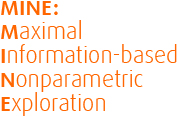# FAQ

We are working hard at making MINE more reliable and easy to use. Before contacting us, please check to see if your question appears on this list, and make sure that you're running the latest version of MINE, since your problem may already be fixed.

### 1. Can you give a a short tutorial on using MINE?

Here's an example to get you going quickly: download MINE, and download the gene expression data set into the same folder. Then type
java -jar MINEv2.jar Spellman.csv 0 -equitability
This will instruct MINE to compare each variable to the 0-th variable (in this case, time) with equitability-oriented parameters.

### 2. How can I generate p-values for the result of a MINE analysis?

Following standard practice, we recommend generating p-values by permuting your data and seeing how likely your observed value of MIC or TIC is to arise from the perturbed data. So, for example, to compute a p-value for, say, an observed TIC of 0.5 computed on data with a sample size of 100, you would take the following steps:

1. Generate N "surrogate" sets of 100 random (x,y) points (for some large N).
2. Compute the TIC scores of all N surrogate sets of data.
3. Calculate an empirical p-value by computing the fraction of the surrogate TIC scores that are greater than or equal to your original observed TIC (in this case, 0.5). E.g., if 0.5 is lower than 8% of the surrogate data TIC scores, then the p-value for a TIC of 0.5 at this sample size is 0.08. Likewise if 0.5 is lower than only 2% of the surrogate data TIC scores, the p-value for a TIC of 0.5 at this sample size is 0.02.

Note that if you are testing the significance of many TIC scores, you will need to correct these p-values to account for multiple testing. We suggest doing so by controlling the false discovery rate as in the original MINE paper.

2. Compute the TIC scores of all N surrogate sets of data.
3. Calculate an empirical p-value by computing the fraction of the surrogate TIC scores that are greater than or equal to your original observed TIC (in this case, 0.5).  E.g., if 0.5 is lower than 8% of the surrogate data TIC scores, then the p-value for a TIC of 0.5 at this sample size is 0.08. Likewise if 0.5 is lower than only 2% of the surrogate data TIC scores, the p-value for a TIC of 0.5 at this sample size is 0.02.
Note that if you are testing the significance of many TIC scores, you will need to correct the above p-values to account for multiple testing.  We suggest doing so by controlling the false discovery rate as in the original MINE paper in Science.  This will require N (number of "surrogate" replicate data sets) to be larger than if you are only computing the p-value of one value of TIC.

### 4. Does MINE work on textual data?

MINE works only on numeric data. If your data set contains text-valued variables, the current version of MINE will ignore those entries and pretend they were blank. You might be able to run MINE effectively by simply replacing each possible value of your text-valued variable with a number (so for instance, if you have "male" and "female" as the possible values of the variable "sex", then you could replace "female" with "1" and "male" with "0"). However, this is not appropriate in all cases.

### 5. What platforms was MINE tested on?

We developed MINE on Windows machines with Java SE 1.7.0_02. It has also been tested on OS X 10.6.8. We cannot guarantee MINE will work on other platforms, though we will do our best to help you out if you believe you have a platform-specific issue.

### 6. When I run MINE in R, I get a java.lang.ClassNotFoundException. How can I fix this?

Despite the information provided in the documentation of the rJava package, we found that changing the slashes in the line that caused the error to periods (so for example: changing '.jnew("data/Dataset"...' to '.jnew("data.Dataset"...') resolves this issue on many platforms.

### 7. When I run MINE, I get a java.lang.OutOfMemoryError. What should I do?

There is a limit (that depends on your computer) to the number of variable pairs MINE will be able to analyze in one go. To overcome this error, split your analysis up into smaller analyses by using, for instance, the -masterVariable option, run the sub-analyses (preferably in parallel), and combine their results. You can also increase the amount of memory that Java allocates to MINE by using Java's -Xmx command-line option.